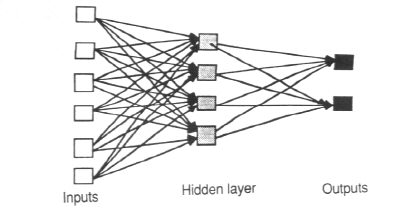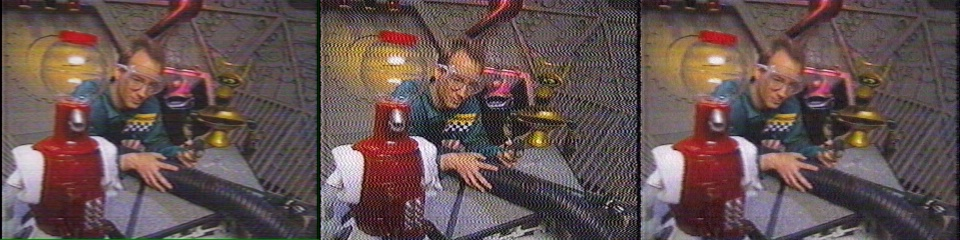# NeuralNet

Author V. C. Mohan
Date Nov 12, 2005

The user must be familiar with Artificial Neural Networks for using this plugin efficientlyNeuralNet has:
A) classic 3 layer classification type neural network with only one output node.In the two functions included the optimization is done differently:
1) NeuralNetBP by usual back propogation
2) NeuralNetRP Resilient Propogation or RPROP (Reidmiller and Braun)
The output of these two functions is either yes (255 ) or No (0)
B)NeuralNetLN :Linear network with a single layer and weights optimized by RPROP method. The output of this is limited to be between 0 and 255.

The plugin has been tested with:
A)NeuralNetBP and NeuralNetRP: greyscale image as input and a corresponding edge detected image wherein edges (above a threshold) were marked as white(255) and non edges as black(0). Training was done using a representative window of this frame. The solution was tested on full frame .
B) NeuralNetLN : An image corrupted by regular freq interference and its 'VFanFilter'-ed output set. Note for high starting weights, results may be inferior.

RP converges faster than BP and so requires fewer iterations. However since the minimization criteria are different, the results can be different. In certain situations one method gives better results than other.

The start frame of input clip must be the frame to be used for training. The training clip must have the processed result corresponding to the start frame of input clip.

Training need to be done in a small window (or along a line) having fully representative cases and having full image amplitude range, as otherwise strange unexpected results may be seen. Time for training Depends upon window size, number of nodes, iterations and 'bestof' value and may take a few seconds to several minutes.

After training, the optimized weights are used for processing all input clip frames. NeuralNet RP and BP outputs all values above a threshold as white, and rest as black.

Facility to monitor error or other diagnostic parameters during training is provided. First output frame will always be a frame with diagnostic plot (and for classification type a histogram of the processed first frame). The diagnostic plot horizontal scale is number of iteration and vertical is scaled parameter value. For Histogram horizontal scale is %age of image y value. The window outline or line used for training is also shown on this plot

For BP and RP if 'test' is true, the input start frame 'sf' is repeatedly processed with NeuralNet solution and the output displayed using threshold values in 'ef' steps from 0 to 100 % with each frame step. If 'ef' is 50 then 50 steps will be used or 2% increase at each frame step occurs

In certain cases after some iterations the error may reach a local minimum, or may start to increase with iteration. Sometimes retraining with a different set of starting weights may correct this problem. 'Bestof' parameter processes with different weight sets and saves the weights which resulted in least error for later use. However this multiplies training time. 'wset' parameter skips weight sets

This plugin works in YUY2, YV12, RGB32 and RGB24 color spaces. Only Red or Luma Y channel is processed.

The input pattern is considered as a grid of 'xpts' * 'ypts'. The output is the value for the center of this grid on the training clip. The input grid is then moved by one pixel and compared with the corresponding output. The training line or window size is to be selected to have sufficient number of cases and so positioned that it encompasses all representative cases.

Incase the training clip was obtained with a grid of x by y, then specifying values other than x and y for xpts and ypts to the network may not be good idea as extraneous data will only add noise and the network may not converge.

Most of the parameters for the three functions are same. While all(except clips) have default values some parameter (training window, hnodes, weight) values need to be specified for better results. Also parameter names may be invariably used for specifying

Parameter Description:-

clip : clip. Clip to be processed No default

clip : clip. Training clip. No default

sf : integer: starting frame number to process on input clip. Default 0

ef : integer: end frame number to process on input clip. Default last frame

tf : integer: frame number on training clip that has proceessed results for 'sf'. default 0

lx : integer: at start frame process limited to a window with left x coordinate. Default 0;

ty : integer: at start frame process limited to a window with top y coordinate. Default 0;

rx : integer: at start frame process limited to a window with right x coordinate. Default frame width - 1;

by : integer: at start frame process limited to a window with bottom y coordinate. Default frame height -1;

elx : integer: at end frame process limited to a window with left x coordinate. Default lx;

ety : integer: at end frame process limited to a window with top y coordinate. Default ty;

erx : integer: at end frame process limited to a window with right x coordinate. Default rx;

eby : integer: at end frame process limited to a window with bottom y coordinate. Default by;

line :boolean: true for training patterns along a line. False for training patterns are in a rectangular window. Default true (line)

tlx : integer: training patterns line / window with left x coordinate. Default lx;

tty : integer: training patterns line / window with top y coordinate. Default ty;

trx : integer: training patterns line / window with right x coordinate. Default rx;

tby : integer: training patterns line / window with bottom y coordinate. Default by;

white: boolean : true if edges are marked white on training clip or false if edges are marked black. Default true (white). NeuralNetLN does not have this parameter.

xpts: integer: Odd number. number of x values in each pattern. default 9. Max xpts X ypts 121

ypts: integer: Odd number : number of y values in each pattern. default 9. Max xpts X ypts 121

hnodes: integer : number of nodes in hidden layer. default sqrt(xnodes X ynodes) 3 to 10. NeuralNetLN does not have this parameter.

iter : integer : number of iterations for training.default 3200 for BP and 200 for RP and 600 for LN

of : float : output weights range will be -0.5 to 0.5 times this value. Default 1.0. NeuralNetLN does not have this parameter.

hf : float : input to hidden layer weights range will be -0.5 to 0.5 times this value. Default 0.0005

activate : string : name of activation sigmoid "logistic", "alogistic", "tanh", "atanh", "gauss", "elliot" and "evero". Default "logistic". NeuralNetLN does not have this parameter.

bestof: integer : trains with this number of different starting weights and selects weights that produced least error sum. Training time increases proportionally.1 to 10 Default 1

wset : integer : skips to this starting weight set without training on them. 1 to 10. Default 1

thresh :integer: %of image value above which all values are considered as object for output. default 60. NeuralNetLN does not have this parameter.

test : boolean : true for test run. False for regular process. Default false NeuralNetLN does not have this parameter.

minstep : float: For BP only. This specifies stepping for quickest descent algorithm. Too small takes too many iterations to converge, too large may be erratic or not converge at all. Default 0.00005

cwt: integer : for BP only:1 to 25. If recognition of all (in this case edges) is more important even if a few non edges are included than missing some edges, then this factor may have higher value say 5. Default 1

plot : string: for RP only: name of diagnostic parameter to be plotted at regular iteration intervals. (output partial derivative)"odedwt", hidden layer partial error derivative "hdedwt", change in output node weights "dwo", change in hidden layer weights "dwh", output weight "owt" , hiddenlayer weights "hwt" and squared error sum "esum". Default "odedwt"

nplot : integer : for RP only: diagnostic plot for node number.Default 0.

Usage examples:-
LoadPlugin("............\Colorit.dll")# this plugin has an edge marker function.Any such can be used
avisource("....")
g=grescale()
t=emarker(g,......)
# test mode with threshold display
NeuralNetBP(g,t,sf=0,ef=99,tlx=0,trx=639,tty=300,tby=312,xpts=3,ypts=3,iter=9000,test=true,wh=0.0005,wo=1.0, minstep=0.00005,cwt=5,bias=1,err=false,nth=300, bestof=1,hnodes=12)
processing mode
NeuralNetBP(g,t,tlx=0,trx=639,tty=300,tby=312,thresh=67,xpts=3,ypts=3,iter=9000,wh=0.0005,wo=1.0, minstep=0.00005,cwt=5,bias=1,hnodes=12)
NeuralNetRP(g,s,tlx=350,trx=550,tty=300,tby=306,thresh=80,xpts=3,ypts=3,iter=150,test=true,plot="esum",nplot =0,wh=0.01,wo=1.0, wset=2,bestof=1, hnodes=3, activate="gauss")
NeuralNetLN(f,i,0,1,tlx=250,trx=450,tty=0,tby=400,xpts=9,ypts=1,iter=600,wh=0.01, wset=1,bestof=1,line=true)

Below is an example of NeuralNetLN. On left is NeuralNetLN output, center is input, on right is the VFAN filtered frame from which a line is used for training.The script used is